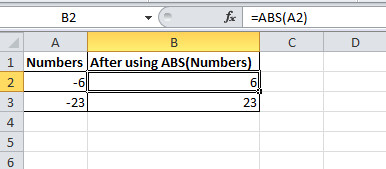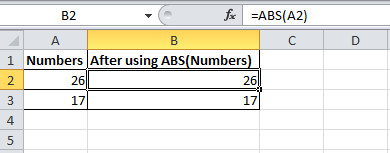# Excel ABS Function To Get Absolute Value With Examples

In this tutorial, learn how to use Excel ABS function to find the absolute value of any number. Get the absolute value of negative as well as a positive number using ABS(). You will learn syntax, uses of various methods and examples for them.

## What is ABS Function in MS Excel

ABS function is the function of Microsoft Excel to return the absolute of any number. With the use of this function, you can convert the negative numbers to positive. It means, if the number contains any sign, the ABS() will give the output of the number without any sign.

However, a positive number will remain positive and there is no effect of the ABS function. This is the built-in function of Excel made as Math/Trigonometric function.

## The syntax of ABS Function in Excel

The function takes a single argument as the positive or negative number to pass. The function can be used in the Excel sheet with the syntax given below.

The above number is the numeric value which can be negative or positive.

## Find the Absolute Value of Negative Number

You can find the absolute value of any negative number in Excel. To perform this task, you have to use the ABS excel function. The function takes a negative number as the argument to pass.

Example: Suppose you want to pass -23 as the argument to ABS function. You have to use `ABS(-23)` to get 23 as the output of the function.

If you want to check it in the excel sheet, you have to see the below-given image. The below image contains the negative numbers in the first column. After using the ABS function on them, you will get the output as given in the second column.The fx in the image showing the `ABS(A2)` with the cell A2 as the argument. The cell A2 contains the number -6 to pass the argument as et the output as positive number 6.

## Get the Absolute Value of Positive Number

In addition to the above all, you can also find the absolute value of the positive number. You have to use the `ABS()` and pass the number as the argument.

Example: Now, let us take an example of a positive number 17 to find the absolute number. You just have to use `ABS(17)` to get the 17 as the output.

You will get the same number in the output for the positive number. See the below-given image showing the column first as the positive number. When you use the ABS function with these numbers. The column second showing the output of the ABS function.The fx showing the same positive number as the argument with the function using. The cell A2 contains the number to pass as the argument to the ABS function.

Hope, you like this post of Excel function ABS to get the absolute number. If you have any query regarding the tutorial, please comment below.

Also tell me, which method you are using to get the absolute value of your number.